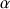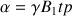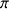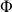# 2D NMR Experiments

### The experiment

When: There is no interaction between the field B1 and µ (they are not in accord) and the precession is kept around the axis Oz.

When: B1 and µ are in accordance. Thus the couple applied by B1 modifies the angleof µ with Oz, and then causes transitions between the magnetic sublevels.

There is resonance. The value of the z magnetization decreases and it appears, in the y direction, the transverse magnetization My.

### The Rotating Coordinate System

Impulsion angleand position of the M vector (Fig. 5) is given by the following relation, with B1 which represents the amplitude or the power of the impulsion and tp the width or duration of the impulsion. It is possible to make these two values vary together in such a way that particularly interesting rotation angles appear. One of these is the angle= 90°.

In this case all the magnetization is orientated in the plane xy and the signal reaches its maximum of intensity. (7)FIG. 5: Location of the M vector after an impulsion time allowing anangle of 90°

An other angle allows the inversion of the M vector by the application of an impulsionor= 180°. In this experiment, the vector M is orientated in –z. Practically, one uses the angle= 45° in order to get a good compromise between measurement time/quality of the response.

### The Free Induction Decay Signal

The signal given by the receiver coil is known under the name of interferogram: Free Induction Decay (FID). In opposition to the continue wavelength signal, the time dependent signal we monitor in this case is an emission signal, because the radiofrequency field B1 is turned off upon the signal acquisition. The experiment gives us practically a variable field which is linear at high frequency following the y axis. In fact it is an oscillator or an emitter with the Larmor frequency of the nuclei under study (7) .Fig. 6: FID made of several superimposed damped sine wave.

The time signal S(t) generated in the receiver coil by the xy component of M weakens under the relaxation process.

### The notion of phase cycle

The phase cycles or phase programs allow to:

• choose the relevant signals and to neglect these ones which do not contain information and which, eventually, are susceptible to hide some other useful signals.
• discriminate the sign of the frequencies in the fl dimension.
• compensate the inhomogeneity from one or several impulsion in the sequence,
• to achieve an optimal quadratic detection in the f2 axis. (5)

Any impulsion in a sequence has its own phase cycle which may be more or less complex. The ideal number of scans must be a multiple of the phase number of the longer cycle. For a better understanding of this method, a standard nomenclature has been defined (Fig. 7).Fig. 7: Standard nomenclature for the phase cycles.

Example 1: The Cyclops Cycle= x,y,-x,-y. 0,1,2,3. This cycle involves the accumulation of a multiple of 4 numbers between each free precession.

The first impulsion B1 occurs around the x and the emission will occur in the y axis, the third impulsion along the - x and the reception in -y, etc.

There are other variations of this cycle like the following:= x,-x, y,-y or 0,2,1,3.
or still:= x,x,-x,-x,y,y,-y,-y or 0,0,2,2,1,1,3,3.

This last one implies a multiple of 8 transient cycles in the accumulation.

### References

1. La détection inverse en RMN ; M Boudonneu Analusis N°1, vol.18, 1990
2. La RMN concepts et méthodes; D. Canet inter éditions 1991
3. La spectroscope de RMN, H. Gunther MASSON 1994.
4. NMR in protein studies. M. P. Williamson. Chem. in Britain April 1991(335-337).
5. RMN 2D BRUKER 1993 Poly. 1, 2 et 3.
6. Resonance Magnétique Nucléaire; Evolution instrumentale et analyse de structure; J.P. Girault le technoscope de biofutur n°38 sept 90
7. Sensitivity enhancement by signal averaging in pulsed by Fourier transform NMR spectroscopy; D. L.Rabenteck; J. of Chem. Ed. Vol. 61, n°70, Oct 1984
8. Sequence Cosy Poly. BRUKER 1993.
9. Topic in chemical instrumentation part 3 and 4. 2 D Methods W. King and K. R. Williams J. of Chem. Educ. vol.67n°4.
10. Topics in chemical instrumentation (Definition) W. King and K. R. Williams
11. Two Dimensional NMR spectroscopy; Applications for chemists and biochemists; W. R. Crousmum, R. M. K. Carlson. Vol 9.
12. Two dimensional NMR Aspect 2000 - 3000 BRUKER
13. G. E. Reeman et Moms, J. ChemSoc, Chem.Comm, 684 (1978).
14. H. Kessler, W. Bernel and C. Giresinger, J. AM. Chem. Soc. 107, 1083 (1985).